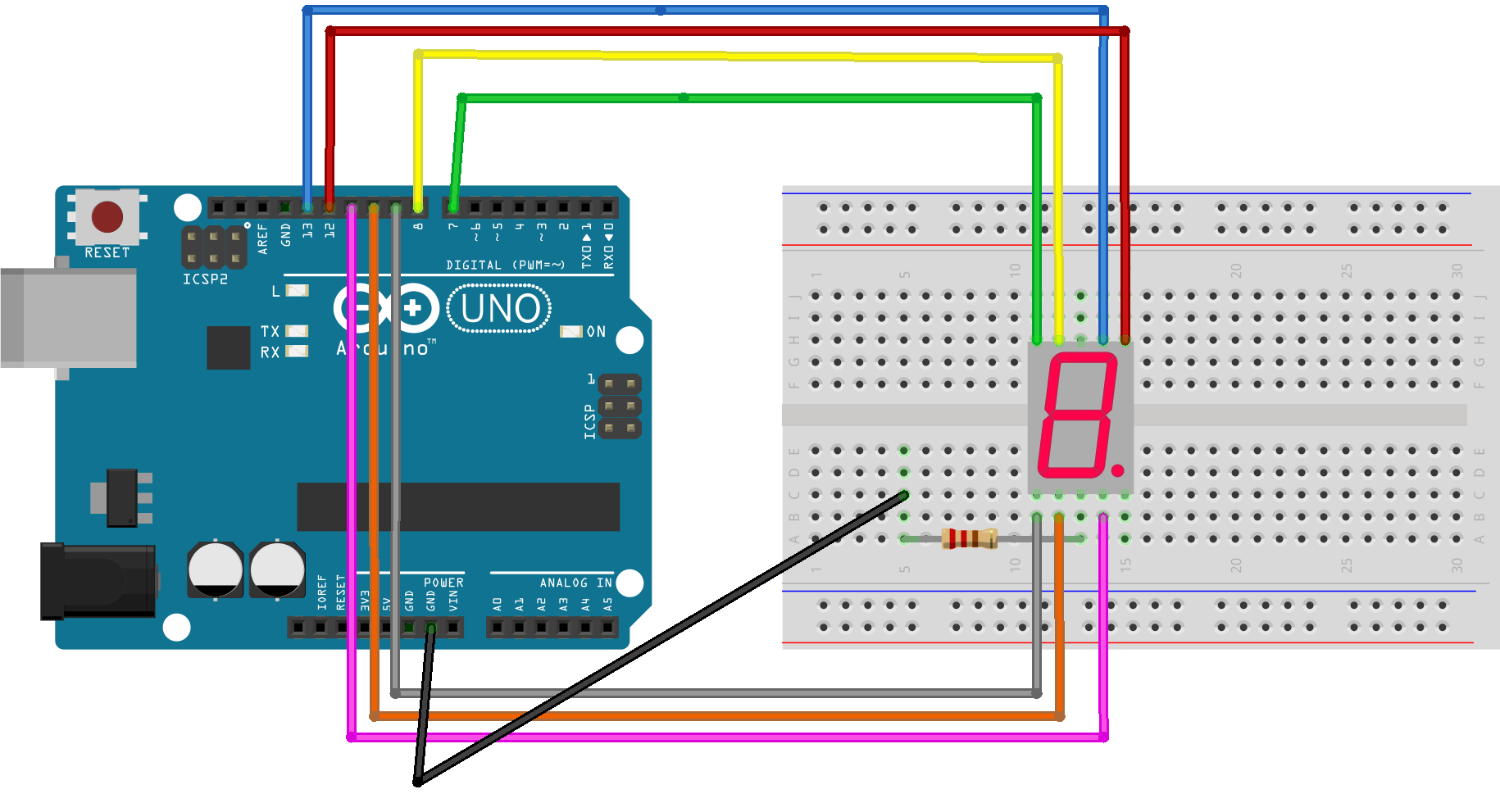# What is Seven Segment Display, how to connect Seven Segment with Arduino and how to create a counter using Seven Segment display and Arduino

 Category: Arduino Tags:Seven Segment Display Code Files

## Introduction

Seven segment displays are widely used in electronic devices, these are user interfacing display devices used to display numeric digit/number. Seven Segment consists of 7 Leds and combination of Leds can display a digit from 0 to 9 as shown in figure below.

We can see in figure we have 7 Leds from a to g and one additional decimal point(dp) Led. We don't need dp led to display a number and we will keep this aside in our further discussion. If we turn on all Led's except g then zero is displayed, if we turn on all Led's eight is displayed similarly we can display any digit from 0 to 1. We have pins in middle to connect to GND or VCC.

## ImplementationFig 2: Arduino with Seven Segment Display

We can use any digital pins of Arduino to connect a-g Leds of Seven Segment in above diagram we used 13-7 pins of Arduino to connect a-g Leds of Seven Segment respectively. We had connected middle wire of Seven Segment with GND so whenever a pin between 13-7 goes high respective Led will turn on. Also we placed 220 ohm resistor between GND pins re reduce current flow else Leds might get burn with current flow. Before building this circuit We will upload below program to our Arduino board.

```int a = 13;
int b = 12;
int c = 11;
int d = 10;
int e = 9;
int f = 8;
int g = 7;

void setup() {
pinMode(a, OUTPUT);
pinMode(b, OUTPUT);
pinMode(c, OUTPUT);
pinMode(d, OUTPUT);
pinMode(e, OUTPUT);
pinMode(f, OUTPUT);
pinMode(g, OUTPUT);
}

void loop() {
Display_0();
delay(1000);
Display_1();
delay(1000);
Display_2();
delay(1000);
Display_3();
delay(1000);
Display_4();
delay(1000);
Display_5();
delay(1000);
Display_6();
delay(1000);
Display_7();
delay(1000);
Display_8();
delay(1000);
Display_9();
delay(1000);
}

void Display_0(){
digitalWrite(a, HIGH);
digitalWrite(b, HIGH);
digitalWrite(c, HIGH);
digitalWrite(d, HIGH);
digitalWrite(e, HIGH);
digitalWrite(f, HIGH);
digitalWrite(g, LOW);
}

void Display_1(){
digitalWrite(a, LOW);
digitalWrite(b, HIGH);
digitalWrite(c, HIGH);
digitalWrite(d, LOW);
digitalWrite(e, LOW);
digitalWrite(f, LOW);
digitalWrite(g, LOW);
}
void Display_2(){
digitalWrite(a, HIGH);
digitalWrite(b, HIGH);
digitalWrite(c, LOW);
digitalWrite(d, HIGH);
digitalWrite(e, HIGH);
digitalWrite(f, LOW);
digitalWrite(g, HIGH);
}
void Display_3(){
digitalWrite(a, HIGH);
digitalWrite(b, HIGH);
digitalWrite(c, HIGH);
digitalWrite(d, HIGH);
digitalWrite(e, LOW);
digitalWrite(f, LOW);
digitalWrite(g, HIGH);
}
void Display_4(){
digitalWrite(a, LOW);
digitalWrite(b, HIGH);
digitalWrite(c, HIGH);
digitalWrite(d, LOW);
digitalWrite(e, LOW);
digitalWrite(f, HIGH);
digitalWrite(g, HIGH);
}
void Display_5(){
digitalWrite(a, HIGH);
digitalWrite(b, LOW);
digitalWrite(c, HIGH);
digitalWrite(d, HIGH);
digitalWrite(e, LOW);
digitalWrite(f, HIGH);
digitalWrite(g, HIGH);
}
void Display_6(){
digitalWrite(a, HIGH);
digitalWrite(b, LOW);
digitalWrite(c, HIGH);
digitalWrite(d, HIGH);
digitalWrite(e, HIGH);
digitalWrite(f, HIGH);
digitalWrite(g, HIGH);
}
void Display_7(){
digitalWrite(a, HIGH);
digitalWrite(b, HIGH);
digitalWrite(c, HIGH);
digitalWrite(d, LOW);
digitalWrite(e, LOW);
digitalWrite(f, LOW);
digitalWrite(g, LOW);
}
void Display_8(){
digitalWrite(a, HIGH);
digitalWrite(b, HIGH);
digitalWrite(c, HIGH);
digitalWrite(d, HIGH);
digitalWrite(e, HIGH);
digitalWrite(f, HIGH);
digitalWrite(g, HIGH);
}
void Display_9(){
digitalWrite(a, HIGH);
digitalWrite(b, HIGH);
digitalWrite(c, HIGH);
digitalWrite(d, HIGH);
digitalWrite(e, LOW);
digitalWrite(f, HIGH);
digitalWrite(g, HIGH);
}```

Program is self explanatory, we just have created 7 variables representing Leds from a to g. Set pinMode as output and Created 10 methods for displaying digits 0 to 9. In loop we have called these methods consecutively in 1 second of delay. Now can can build circuit as shown in figure and plug a 5-10v battery to turn on Seven Segment Display. The display will show a counter from 0-9.

To see output of this circuit you can watch video here:

 Like0 PeopleNikhil Joshi Ceo & Founder at Dotnetlovers Atricles: 158 Questions: 16 Given Best Solutions: 16 *

Login via:x